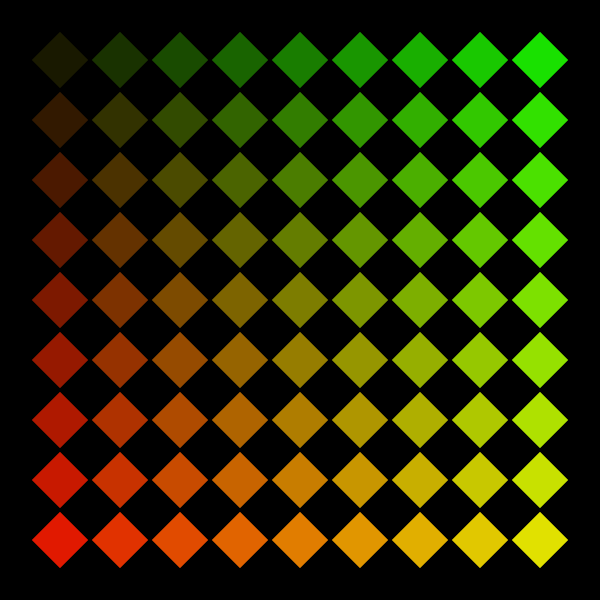# p5: Transformation Matrix (rotate, translate, scale)Check this tutorial

In brief always follow this order:

1. push
2. translate first to the pivot, the center of rotation
3. rotate (don’t forget to convert in radians)
4. draw everything as if the origin (the center of your composition) was 0, 0
5. pop

A longer explanation here.

Transformations and for loops:```var rows = 10;
var cols = 10;
var distanceX = 30;
var distanceY = 30;

function setup() {
createCanvas(300, 300);
background(0);
rectMode(CENTER);

noStroke();
for (var r = 1; r < rows; r++) {
for (var c = 1; c < cols; c++) {
push();
translate(c * distanceX, r * distanceY);
fill(r * 25, c * 25, 0);
rect(0, 0, 20, 20);
pop();
}
}
}
```

### Exercise

Now try to reproduce the plotter drawing below (or your take on it). (Georg Nees Schotter, 1968)